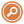# How Are Histograms Used in Statistics?A histogram chart is a graphical representation of the distribution of data. The distribution of data is the way in which the data is spread out. The histogram will show the frequency of data points within a certain range. The histogram is used in statistics to help identify the distribution of data and to help find the median, mode, and range of the data. Below, we will further explore this important data visualization tool.

## What is a histogram used for?

Histograms can be used to compare the distributions of two or more sets of data. They can also be used to determine the distribution of a set of data by looking at the shape of the histogram and the location of the median and the mode.

Additionally, the histogram can identify the center of a distribution, the spread of a distribution, and the shape of a distribution. The center of a distribution is the location where the majority of the data is located. The spread of a distribution is the distance from the center to the outermost points of the distribution. The shape of a distribution is determined by the height of the bars in the histogram and the shape of the curve.

## How do you interpret histograms?

There are several ways to interpret histograms:

1. The shape of the histogram can give you an idea of the distribution of the data.

2. The center of the histogram can give you an idea of the typical value of the data.

3. The spread of the histogram can give you an idea of the variability of the data.

4. The shape and spread of the histogram can give you an idea of how the data is distributed.

The taller the histogram, the more data there is within that interval. The wider the histogram, the more variation there is in the data. The bars on a histogram can help you see the distribution of a data set. You can use this information to make decisions about how to collect data, how to analyze the data, and how to present the data.

## How do you create a histogram?

To make a histogram, whether by hand or in a program like Excel, you first need to collect data. This data can be anything, from the number of people in your city to the number of times you’ve been to the gym this year. Once you have your data, you need to decide what range to use for the horizontal axis of your histogram. This range will be determined by the highest and lowest numbers in your data set.

Once you have your range, you need to divide it into intervals. The intervals will be uniform, meaning that each interval will be the same size. To create these intervals, you will need to determine the width of each interval. The width of each interval will be the size of the range divided by the number of intervals.

Now that you have your intervals, you can begin to graph your data. Draw a horizontal line across the middle of your first interval. This line will represent the median of your data set. Then, draw a vertical line up from the median to the top of the first interval. This line will represent the first quartile of your data set. Finally, draw a vertical line down from the median to the bottom of the first interval. This line will represent the third quartile of your data set.

Now that you have your first quartile, median, and third quartile, you can begin to fill in your histogram. Draw a bar above the median that extends to the first quartile. This bar will represent the upper quartile of your data set. Then, draw a bar below the median that extends to the third quartile. This bar will represent the lower quartile of your data set. Finally, draw a bar above the first quartile and below the third quartile. This bar will represent the range of your data set.

You can continue to fill in your histogram by drawing bars for each of the intervals. Be sure to label each bar with the frequency of data that it represents.

Overall, histograms are a useful statistical tool that helps visualize a data set, find the shape of the distribution, and find the center and spread of the data.

Check the comment section below for additional information, share what you know, or ask a question about this article by leaving a comment below. And, to quickly find answers to your questions, use our searchengine.

Note: Some of the information in samples on this website may have been impersonated or spoofed.